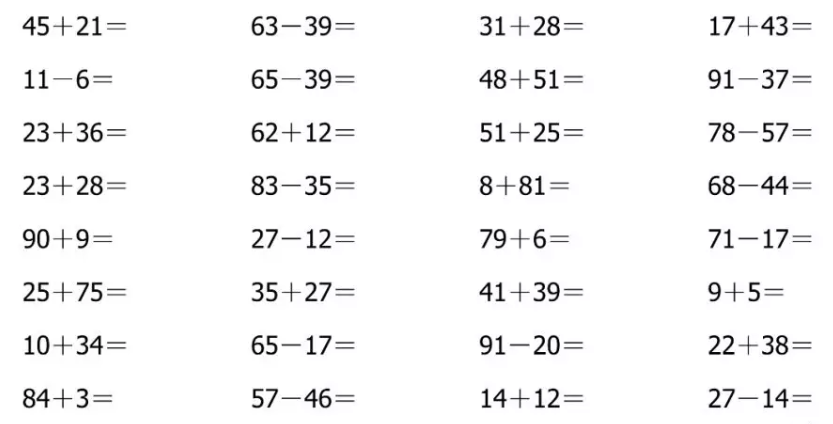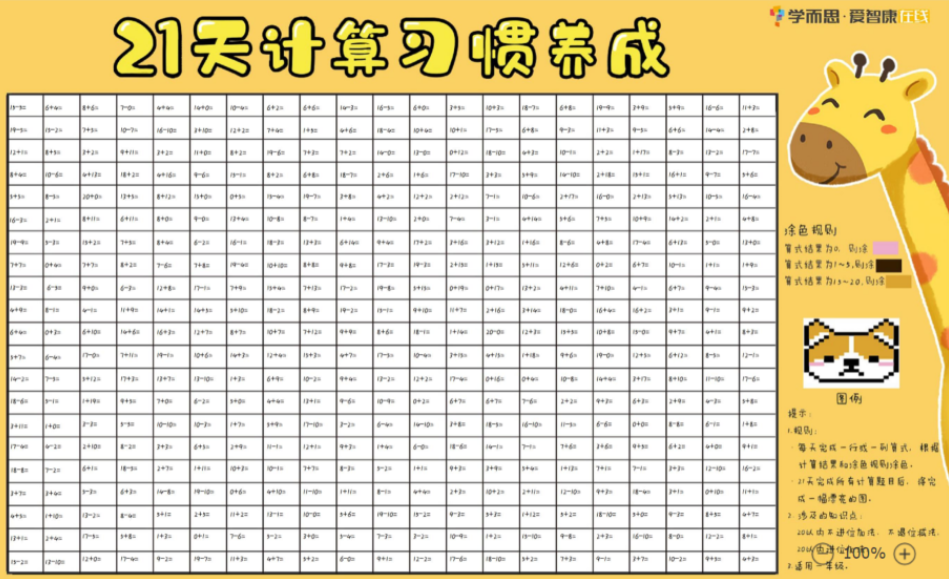# 小学一年级至二年级数学计算题

2019-11-24 23:50:02 　来源：网络整理福利资料包大放送_《21天计算习惯养成》大海报pdf版免费领取（分一年级/二年级版）另外学而思爱智康的老师还为大家精心整理了：

《21天计算习惯养成》大海报pdf版

助你效率翻倍！取得优异成绩！

部分资料截图如下：1.一个算式里有括号，要先算括号里面的，再算括号外面的。

2.一个算式里没有括号，从左到右，依次计算。

24+(8-4)=____　　23+(9-8)=____　　40-(2+8)=____

32-(4+6)=____　　72-(9-5)=____　　66-(4+6)=____

39-(4+5)=____　　89-(7-5)=____　　63+(3+7)=____

8+(50-6)=____　　4+(23+42)=____　　60+(30-7)=____

24+(8-4)=28　　23+(9-8)=24　　40-(2+8)=30

32-(4+6)=22　　72-(9-5)=68　　66-(4+6)=56

39-(4+5)=30　　89-(7-5)=86　　63+(3+7)=73

8+(50-6)=52　　4+(23+42)=69　　60+(30-7)=83QQ扫一扫您将获得群内福利家长互动海量资料同步免费视频课程了解最新信息及时解读资讯你可能感兴趣的文章立即咨询1对1在线课程*学而思爱智康会在24小时内与您取得电话联系大家都在看热门课程介绍限时免费领取学习相关10月13日初中入学规划KET/PET备考

• 全国课程在线咨询
• 咨询热线：4000-121-121
• 扫描注册有礼

• 让学习更有效